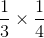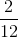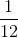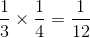ISEE Lower Level Quantitative : Operations with fractions and whole numbers

Example Questions

← Previous 1 3 4 5 6 7 8 9 18 19

Example Question #2 : Multiply A Fraction Or Whole Number By A Fraction: Ccss.Math.Content.5.Nf.B.4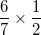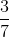Explanation:

When we multiply fractions, we multiply the numerator by the numerator and the denominator by the denominator.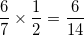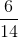can be reduced by dividing both sides by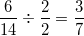Example Question #3 : Multiply A Fraction Or Whole Number By A Fraction: Ccss.Math.Content.5.Nf.B.4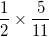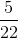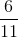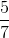Explanation:

When we multiply fractions, we multiply the numerator by the numerator and the denominator by the denominator.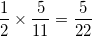Example Question #1 : Operations With Fractions And Whole Numbers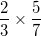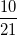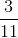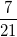Explanation:

When we multiply fractions, we multiply the numerator by the numerator and the denominator by the denominator.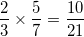Example Question #5 : Multiply A Fraction Or Whole Number By A Fraction: Ccss.Math.Content.5.Nf.B.4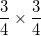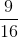Explanation:

When we multiply fractions, we multiply the numerator by the numerator and the denominator by the denominator.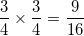Example Question #1053 : Numbers And Operations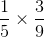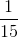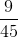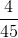Explanation:

When we multiply fractions, we multiply the numerator by the numerator and the denominator by the denominator.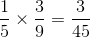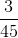can be reduced by dividing both sides by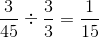Example Question #1054 : Numbers And Operations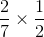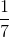Explanation:

When we multiply fractions, we multiply the numerator by the numerator and the denominator by the denominator.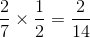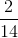can be reduced by dividing both sides by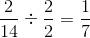Example Question #1061 : Numbers And Operations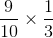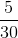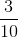Explanation:

When we multiply fractions, we multiply the numerator by the numerator and the denominator by the denominator.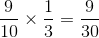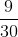can be reduced by dividing both sides by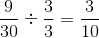Example Question #1062 : Numbers And Operations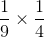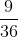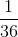Explanation:

When we multiply fractions, we multiply the numerator by the numerator and the denominator by the denominator.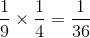Example Question #1063 : Numbers And Operations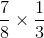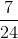Explanation:

When we multiply fractions, we multiply the numerator by the numerator and the denominator by the denominator.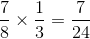Example Question #2 : Operations With Fractions And Whole Numbers